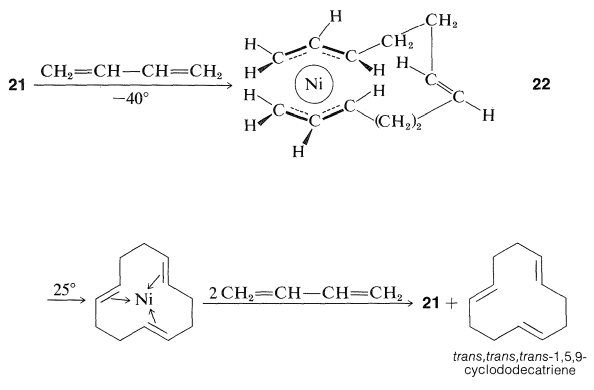# 31.5: π-Propenyl Complexes of Nickel

A considerable body of highly useful chemistry based on nickel has been developed, largely by the German chemist, G. Wilke. Many of these reactions involve what are called $$\pi$$-propenyl ($$\pi$$-allyl) complexes and their formation has a close analogy in the formation of ferrocene from cyclopentadienylmagnesium compounds and ferric chloride (Section 31-1). Treatment of $$\ce{NiBr_2}$$ with two moles of 2-propenylmagnesium bromide gives a stable (albeit oxygen sensitive) substance of composition $$\ce{(C_3H_5)_2Ni}$$:Unlike $$\ce{C_3H_5MgBr}$$, the metal compound has a very complex proton NMR spectrum. Analysis of the spectrum indicates it arises from a mixture (75:25) of two $$\ce{(C_3H_5)_2Ni}$$ isomers with each isomer having its $$\ce{C_3H_5}$$ groups in a rigid planar arrangement as follows:These facts can be accommodated by the trans- and cis-di-$$\pi$$-propenylnickel structures, $$20$$. Di-$$\pi$$-propenylnickel has many interesting reactions, among which are the following examples:The $$\pi$$-propenyl-type structures are more stable for nickel than for other metals such as iron. With 1,3-butadiene, $$\ce{Fe(CO)_5}$$ forms a double $$\pi$$ complex, whereas $$\ce{Ni(CO)_4}$$ produces a bis-$$\pi$$-propenyl-type structure, $$21$$:With more 1,3-butadiene, $$21$$ is converted first to $$22$$, which after rearrangement reacts with 1,3-butadiene to give back $$21$$ with liberation of trans,trans,trans-1,5,9-cyclododecatriene:The overall sequence thus provides a catalytic route for the cyclic trimerization of 1,3-butadiene.

Ethene and alkynes react with $$21$$ in the presence of excess carbon monoxide to give ten-membered ring compounds, whereas the reaction of $$21$$ with excess carbon monoxide results in formation of a mixture of six- and eight-membered rings: